# Action and Reaction Newtons 3 rd Law of

• Slides: 11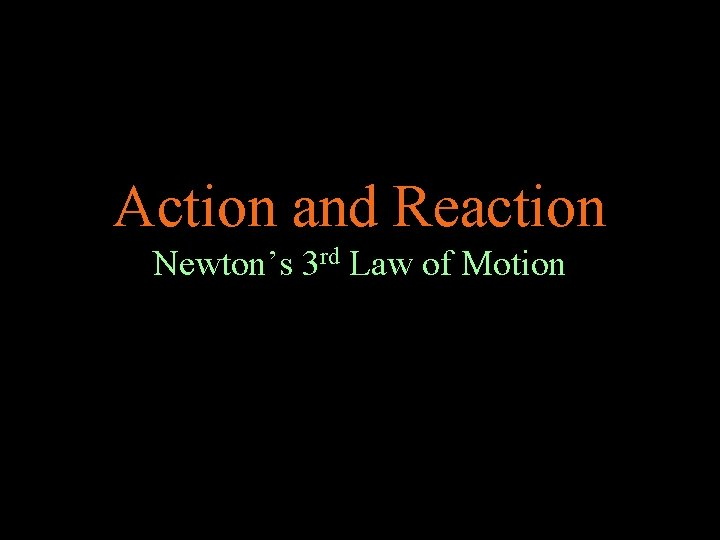Action and Reaction Newton’s 3 rd Law of Motion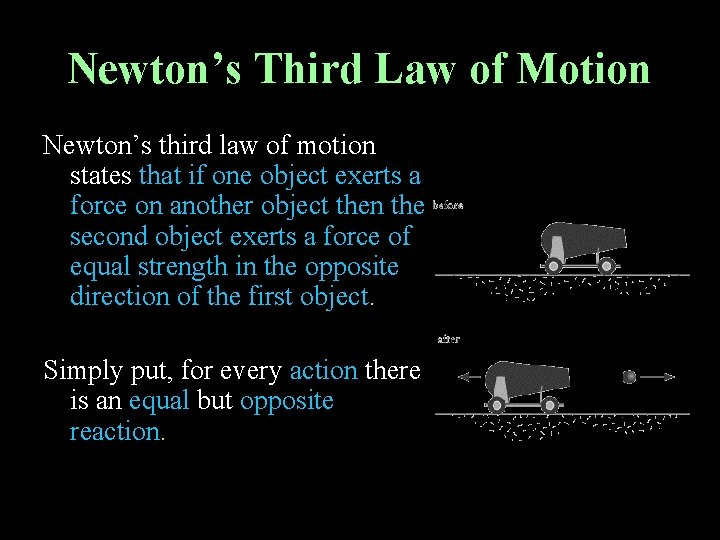Newton’s Third Law of Motion Newton’s third law of motion states that if one object exerts a force on another object then the second object exerts a force of equal strength in the opposite direction of the first object. Simply put, for every action there is an equal but opposite reaction.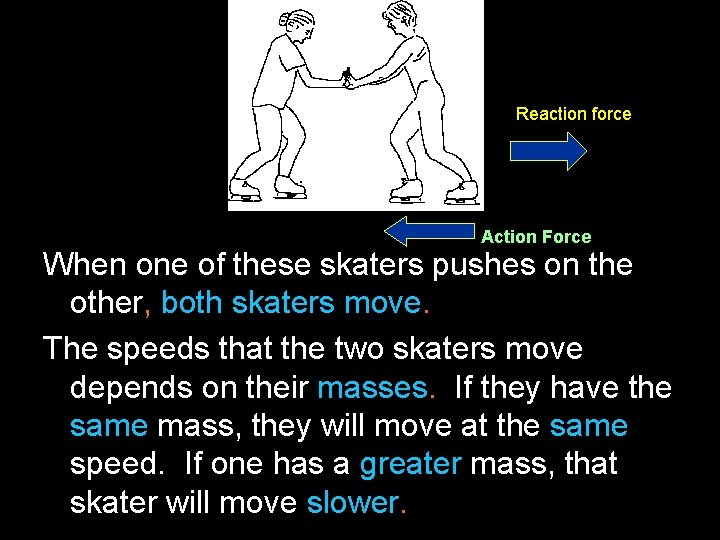Reaction force Action Force When one of these skaters pushes on the other, both skaters move. The speeds that the two skaters move depends on their masses. If they have the same mass, they will move at the same speed. If one has a greater mass, that skater will move slower.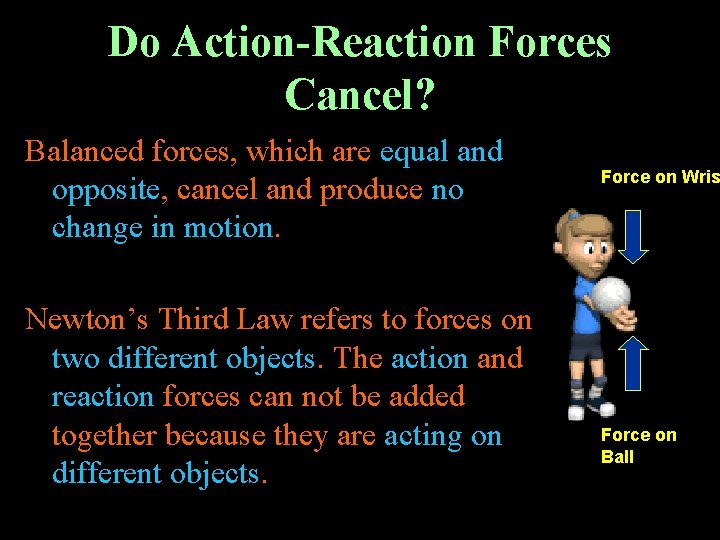Do Action-Reaction Forces Cancel? Balanced forces, which are equal and opposite, cancel and produce no change in motion. Newton’s Third Law refers to forces on two different objects. The action and reaction forces can not be added together because they are acting on different objects. Force on Wris Force on Ball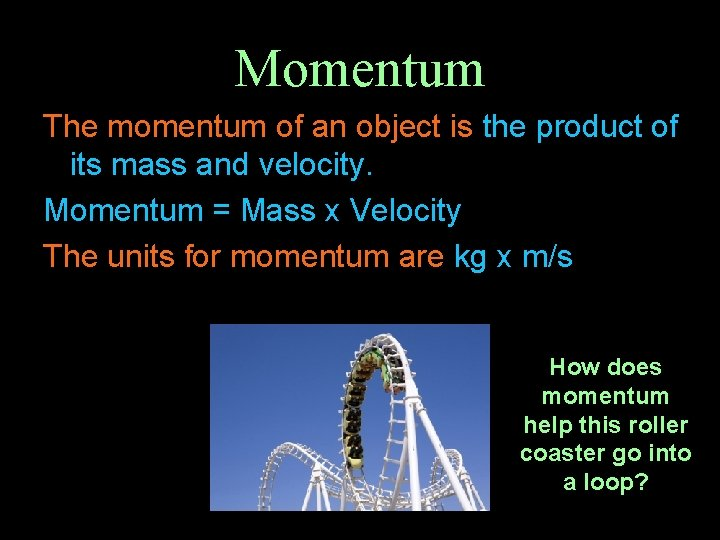Momentum The momentum of an object is the product of its mass and velocity. Momentum = Mass x Velocity The units for momentum are kg x m/s How does momentum help this roller coaster go into a loop?Momentum The momentum of an object is in the same direction as its velocity. The more momentum an object has the harder it is to stop. Which object would be the hardest to stop?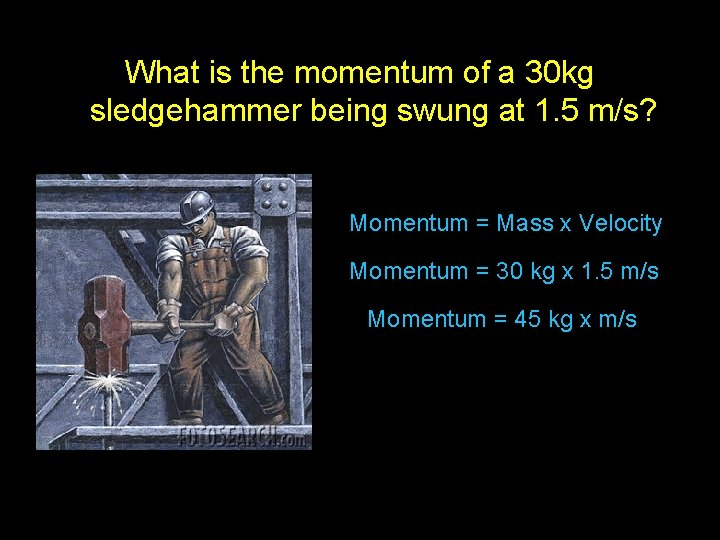What is the momentum of a 30 kg sledgehammer being swung at 1. 5 m/s? Momentum = Mass x Velocity Momentum = 30 kg x 1. 5 m/s Momentum = 45 kg x m/sConservation of Momentum The law of conservation of momentum states that the total momentum of the objects that interact does not change. The total momentum of any group of objects remains the same unless outside forces act on the objects.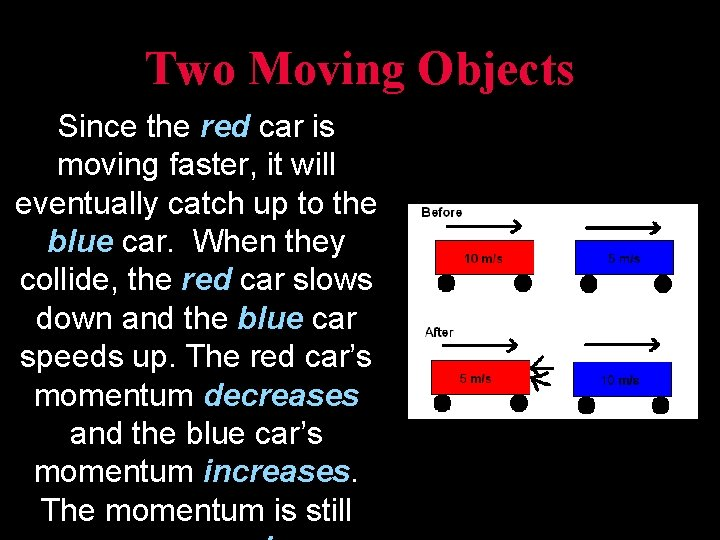Two Moving Objects Since the red car is moving faster, it will eventually catch up to the blue car. When they collide, the red car slows down and the blue car speeds up. The red car’s momentum decreases and the blue car’s momentum increases. The momentum is still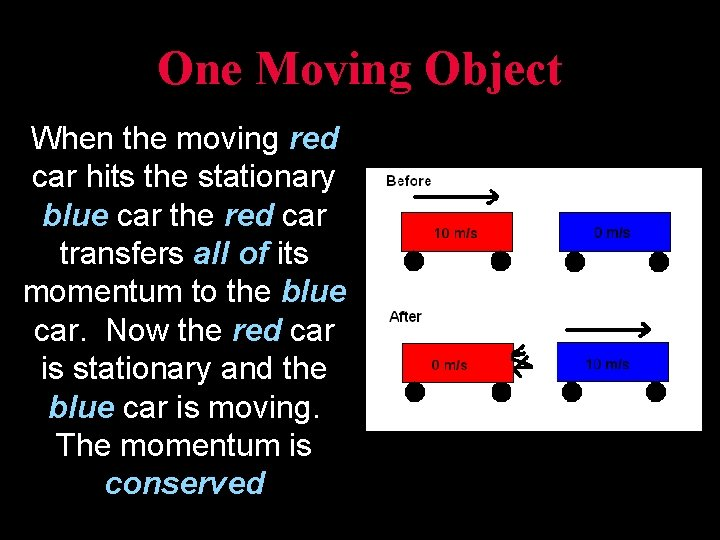One Moving Object When the moving red car hits the stationary blue car the red car transfers all of its momentum to the blue car. Now the red car is stationary and the blue car is moving. The momentum is conserved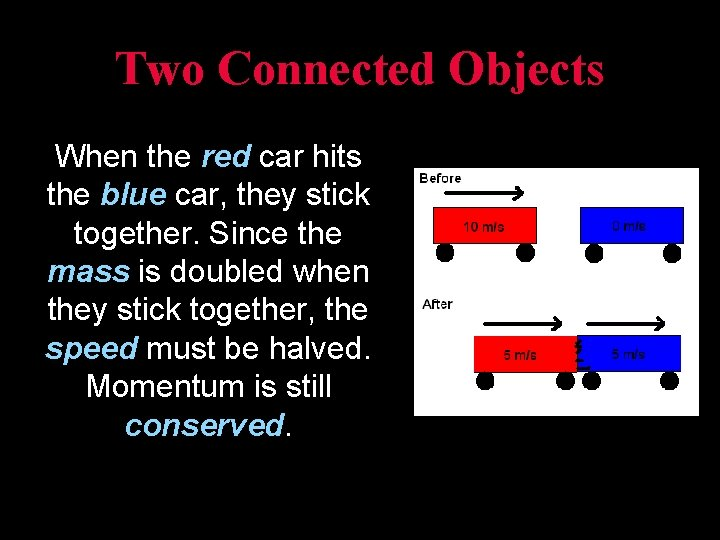Two Connected Objects When the red car hits the blue car, they stick together. Since the mass is doubled when they stick together, the speed must be halved. Momentum is still conserved.